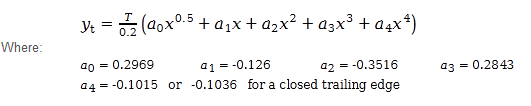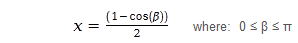Airfoil Tools
Search 1636 airfoilsYour Reynold number range is 50,000 to 1,000,000. (set)

# NACA 4 digit airfoil generator (NACA 0017 AIRFOIL)

### Dat file

```NACA 0017 Airfoil M=0.0% P=0.0% T=17.0%
1.000000  0.001785
0.996057  0.002567
0.984292  0.004877
0.964888  0.008616
0.938153  0.013627
0.904508  0.019712
0.864484  0.026647
0.818712  0.034192
0.767913  0.042099
0.712890  0.050112
0.654508  0.057966
0.593691  0.065377
0.531395  0.072044
0.468605  0.077648
0.406309  0.081868
0.345492  0.084398
0.287110  0.084972
0.232087  0.083394
0.181288  0.079560
0.135516  0.073472
0.095492  0.065236
0.061847  0.055050
0.035112  0.043170
0.015708  0.029875
0.003943  0.015419
0.000000  0.000000
0.003943 -0.015419
0.015708 -0.029875
0.035112 -0.043170
0.061847 -0.055050
0.095492 -0.065236
0.135516 -0.073472
0.181288 -0.079560
0.232087 -0.083394
0.287110 -0.084972
0.345492 -0.084398
0.406309 -0.081868
0.468605 -0.077648
0.531395 -0.072044
0.593691 -0.065377
0.654508 -0.057966
0.712890 -0.050112
0.767913 -0.042099
0.818712 -0.034192
0.864484 -0.026647
0.904508 -0.019712
0.938153 -0.013627
0.964888 -0.008616
0.984292 -0.004877
0.996057 -0.002567
1.000000 -0.001785
```
 Max Camber (%) First digit. 0 to 9.5% Max camber position (%) Second digit. 0 to 90% Thickness (%) Third & fourth digit. 1 to 40% Number of points 20 to 200 Cosine spacing Cosine or linear spacing Close Trailing edge Open or closed TE
 NACA 4 digit airfoils in the database NACA 0006 NACA 0008 NACA 0009 NACA 0010 NACA 0012 NACA 0015 NACA 0018 NACA 0021 NACA 0024 NACA 1408 NACA 1410 NACA 1412 NACA 2408 NACA 2410 NACA 2411 NACA 2412 NACA 2414 NACA 2415 NACA 2418 NACA 2421 NACA 2424 NACA 4412 NACA 4415 NACA 4418 NACA 4421 NACA 4424 NACA 6409 NACA 6412

## NACA 4 digit airfoil specification

This NACA airfoil series is controlled by 4 digits e.g. NACA 2412, which designate the camber, position of the maximum camber and thickness. If an airfoil number is
NACA MPXX
e.g.
NACA 2412
then:
• M is the maximum camber divided by 100. In the example M=2 so the camber is 0.02 or 2% of the chord
• P is the position of the maximum camber divided by 10. In the example P=4 so the maximum camber is at 0.4 or 40% of the chord.
• XX is the thickness divided by 100. In the example XX=12 so the thiickness is 0.12 or 12% of the chord.

## NACA 4 digit airfoil calculation

The NACA airfoil section is created from a camber line and a thickness distribution plotted perpendicular to the camber line.

The equation for the camber line is split into sections either side of the point of maximum camber position (P). In order to calculate the position of the final airfoil envelope later the gradient of the camber line is also required. The equations are:The thickness distribution is given by the equation:• The constants a0 to a4 are for a 20% thick airfoil. The expression T/0.2 adjusts the constants to the required thickness.
• At the trailing edge (x=1) there is a finite thickness of 0.0021 chord width for a 20% airfoil. If a closed trailing edge is required the value of a4 can be adjusted.
• The value of yt is a half thickness and needs to be applied both sides of the camber line.

Using the equations above, for a given value of x it is possible to calculate the camber line position Yc, the gradient of the camber line and the thickness. The position of the upper and lower surface can then be calculated perpendicular to the camber line.The most obvious way to to plot the airfoil is to iterate through equally spaced values of x calclating the upper and lower surface coordinates. While this works, the points are more widely spaced around the leading edge where the curvature is greatest and flat sections can be seen on the plots. To group the points at the ends of the airfoil sections a cosine spacing is used with uniform increments of β